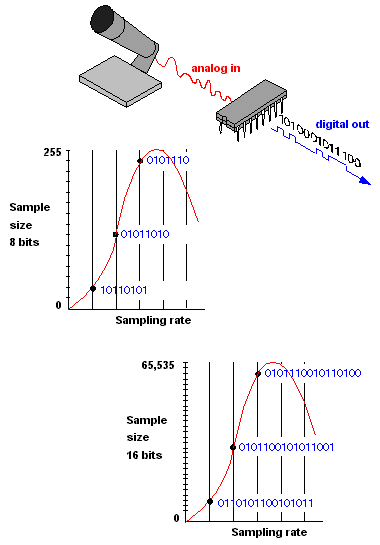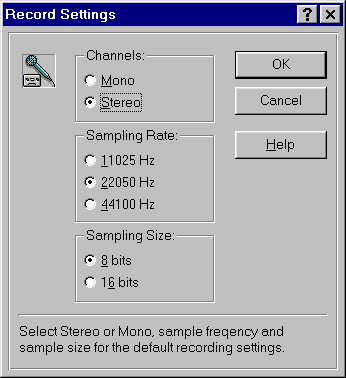# ANALOGUE TO DIGITAL SAMPLING TECHNIQUES

Filed under: DIGITAL AUDIO RECORDING,KNOWLEDGEMENT,Tak Berkategori — Tag: , — KING JAZZ (Bayu Wirawan) @ 09.48
SAMPLING TECHNIQUE

(1) In statistics, the analysis of a group by determining the characteristics of a significant percentage of its members chosen at random.

(2) Converting analog signals into digital form. Audio and other analog signals are continuous waveforms that are analyzed at various points in time and converted into digital samples. The accuracy with which the digital samples reflect their analog origins is based on “sampling rate” and “sample size.” See A/D converter.

Sampling Rate – When to Measure
The sampling rate is the number of times per second that the waveform is measured, which typically ranges from 8 to 192 thousand times per second (8 kHz to 192 kHz). The greater the rate, the higher the frequency that can be captured. For a comparison of high-quality samples, see high-resolution sampling rates.

The sampling rate must be at least twice that of the analog frequency being captured. For example, the sampling rate used to create the digital data on a CD is 44.1 kHz, slightly more than double the 20kHz frequency an average person can hear. The sampling rate for digitizing voice for a toll-quality conversation is typically 8,000 times per second (8 kHz), twice the 4 kHz required for the full spectrum of the human voice. See analog and Nyquist theorem.

Sample Size – The Measurement
Also called “resolution” and “precision,” the sample size is the measurement of each sample point on a numeric scale. Known as “quantizing,” the sample point is turned into the closest whole number. The more granular the scale (the more increments), the more accurate the digital sample represents the original analog signal. See oversamplingquantization and PCM.

Sampling SoundThe faster the sampling rate and the larger the sample size, the more accurately sound can be digitized. An 8-bit sample breaks the sound wave into 255 increments compared with 65,535 for a 16-bit sample.
Sampling DialogThis recording dialog from an earlier Sound Blaster sound card shows typical sampling options for digitizing sound into Windows WAV files.

Taken From: http://encyclopedia2.thefreedictionary.com/Digital+sampling